• 使用偏最小二乘结构方程模型来确定学生的性格，对学习环境的感知，学习方法以及环境对学生的影响对人类尸体解剖室中本科生专业学生活动的影响。 这是对以前从本科生卫生专业学生进行的横断面调查收集的数据的二次...
• 为克服大坝位移预测回归模型中，选取因子的多重相关性对回归方程的影响，同时考虑到位移的波动性对残差的影响，提出一种偏最小二乘-马尔科夫链大坝位移预测模型最小二乘法能够有效解决多重相关性的问题，...
• %建立偏最小二乘回归模型 %(1)提取所有可能的主成分 X=data(:,1:5); Y=data(:,6:8); E0=stand(X) F0=stand(Y) A=rank(E0) [W,C,T,U,P,R]=plspcr(E0,F0)%提取所有可能的主成分 %（2）主成分解释能力分析 %计算主成分...
clear;
%多重相关性诊断
cr=corrcoef(data) %计算变量之间的相关系数
%建立偏最小二乘回归模型
%(1)提取所有可能的主成分
X=data(:,1:5);
Y=data(:,6:8);
E0=stand(X)
F0=stand(Y)
A=rank(E0)
[W,C,T,U,P,R]=plspcr(E0,F0)%提取所有可能的主成分

%（2）主成分解释能力分析
%计算主成分累计复测定系数
RA=plsra(T,R,F0,A)
%计算主成分的信息解释能力
[Rdx,RdX,RdXt,Rdy,RdY,RdYt]=plsrd(E0,F0,T,A)

%（3）考查第一主成分间的相关性
%绘制t1/u1图直观的考查第一主成分间的相关性
cr=plsutcor(U,T)

%（4）求PLS回归方程的系数
%先求标准化因变量关于主成分t1的经验回归系数
TCOEFF=R(:,1)%这组系数存于plspcr函数的最后一个输出变量R中
%再求标准化因变量关于标准化自变量的经验回归系数
SCOEFF=pls(1,5,W,P,R)%1表示用于建模的主成分个数，5表示自变量个数
%最后求原始因变量关于原始自变量的经验回归系数
[COEFF,INTERCEP]=plsiscoeff(X,Y,SCOEFF)%对标准化的回归系数进行逆标准化处理，输出原始自变量对因变量的回归系数及常数项

%（5）变量投影重要性分析与模型的改进
VIP=plsvip(W,RdY,RdYt,1)%得出值表示第j个自变量对因变量的解释能力，若较小，则删除该自变量重新建模

展开全文• 偏最小二乘回归与AR模型组合预测北京市年生活用水量，顾月红，，本文使用偏最小二乘回归模型确定北京市年生活用水量和挑选的5项影响因子之间的回归方程，发现该市年生活用水量和提前3年的各项因�
• 1 偏最小二乘回归方程偏最小二乘回归分析建模的具体步骤 模型效应负荷量 交叉有效性检验 在实际问题中，经常遇到需要研究两组多重相关变量间的相互依赖关系，并研究用 一组变量（常称为自变量或预...
偏最小二乘回归系列博文：

偏最小二乘回归（一）：模型介绍

偏最小二乘回归（二）：一种更简洁的计算方法

偏最小二乘回归（三）：身体特征与体能训练结果的 案例分析

目录

1 偏最小二乘回归方程式

偏最小二乘回归分析建模的具体步骤

模型效应负荷量

交叉有效性检验

在实际问题中，经常遇到需要研究两组多重相关变量间的相互依赖关系，并研究用 一组变量（常称为自变量或预测变量）去预测另一组变量（常称为因变量或响应变量）， 除了最小二乘准则下的经典多元线性回归分析（MLR），提取自变量组主成分的主成分回归分析（PCR）等方法外，还有近年发展起来的偏最小二乘（PLS）回归方法。

偏最小二乘回归提供一种多对多线性回归建模的方法，特别当两组变量的个数很 多，且都存在多重相关性，而观测数据的数量（样本量）又较少时，用偏最小二乘回归建立的模型具有传统的经典回归分析等方法所没有的优点。 偏最小二乘回归分析在建模过程中集中了主成分分析，典型相关分析和线性回归分 析方法的特点，因此在分析结果中，除了可以提供一个更为合理的回归模型外，还可以 同时完成一些类似于主成分分析和典型相关分析的研究内容，提供更丰富、深入的一些 信息。

本章介绍偏最小二乘回归分析的建模方法；通过例子从预测角度对所建立的回归模 型进行比较。

1 偏最小二乘回归方程式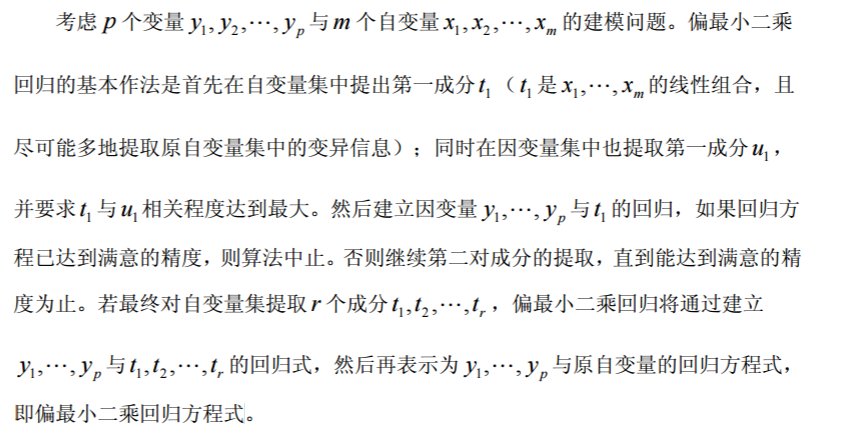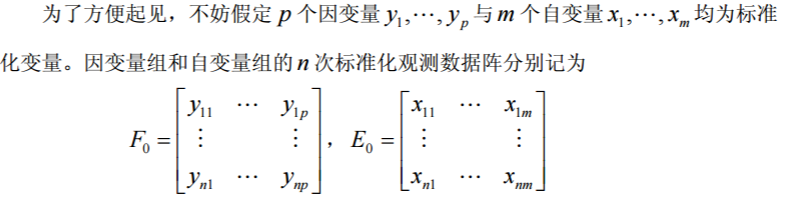偏最小二乘回归分析建模的具体步骤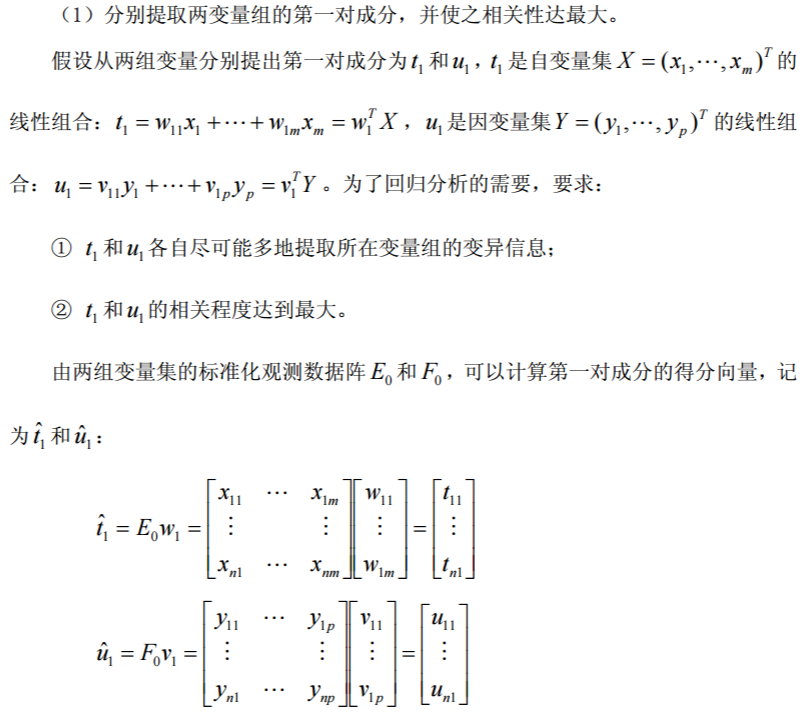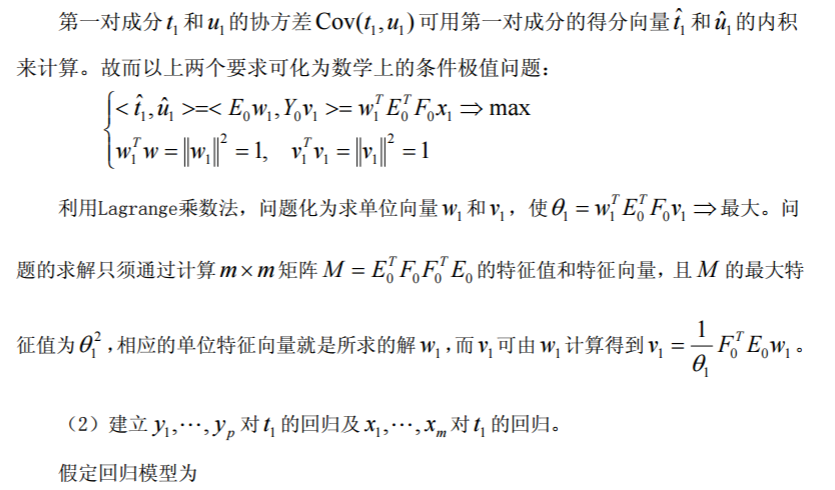模型效应负荷量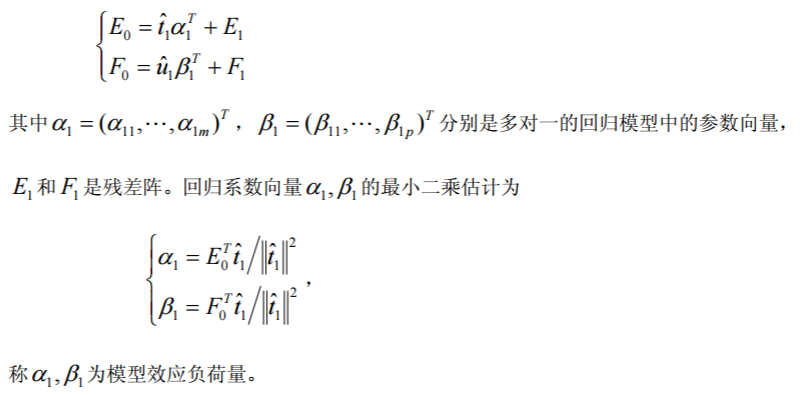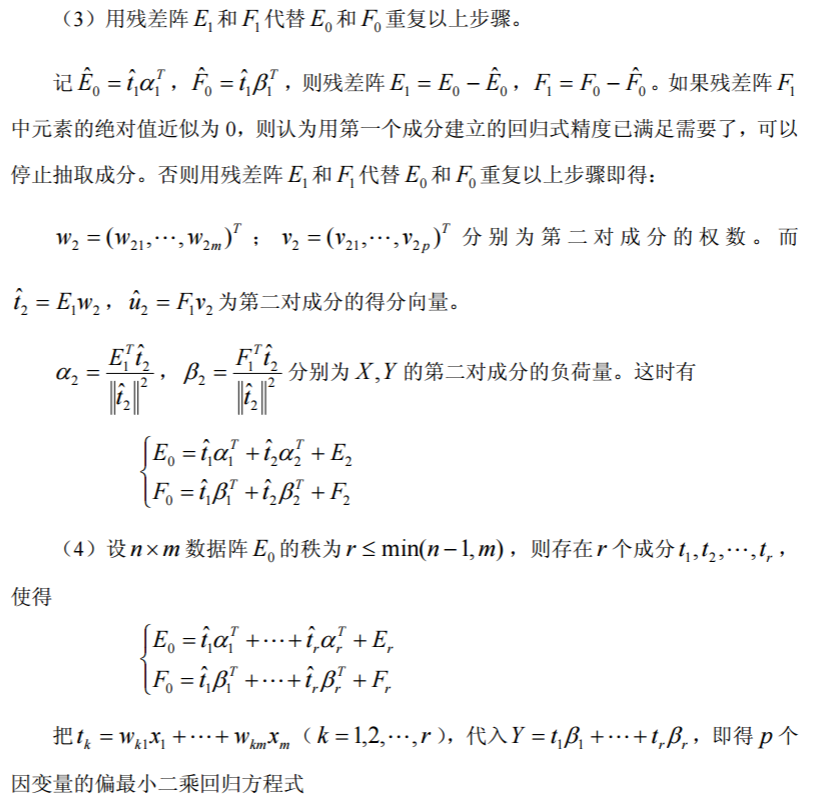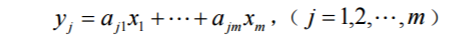（5）交叉有效性检验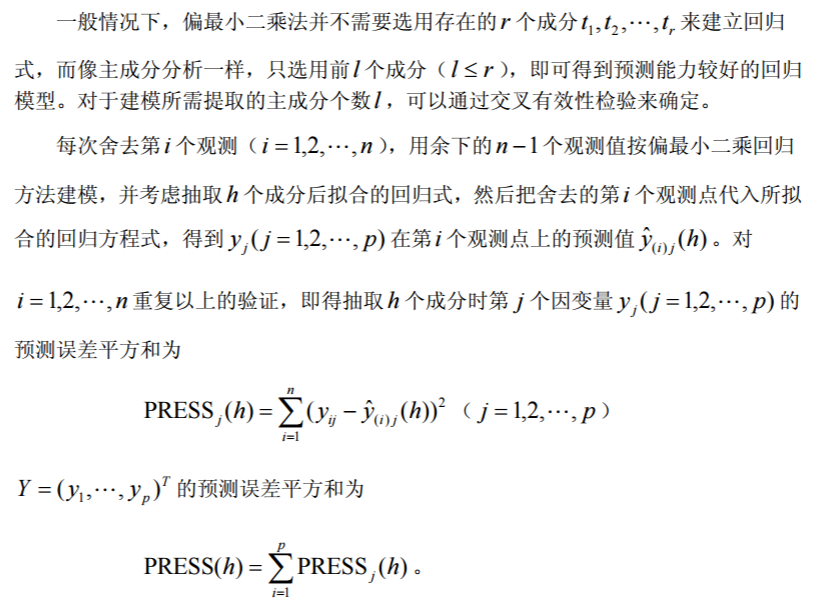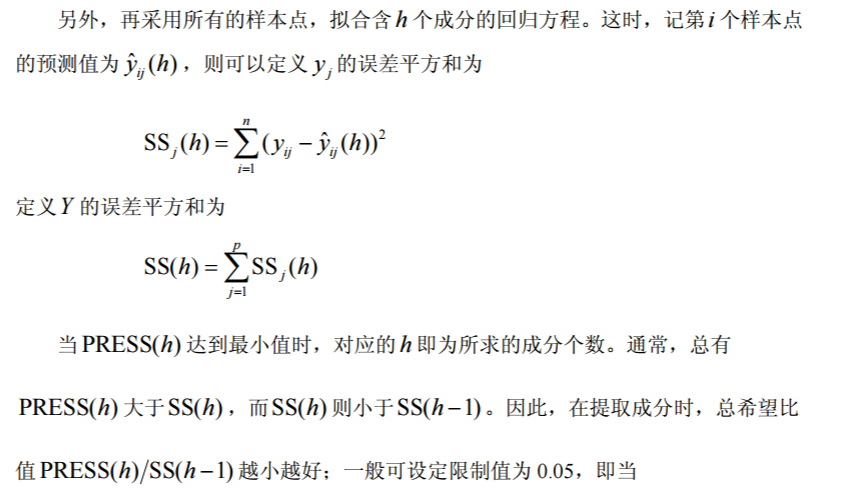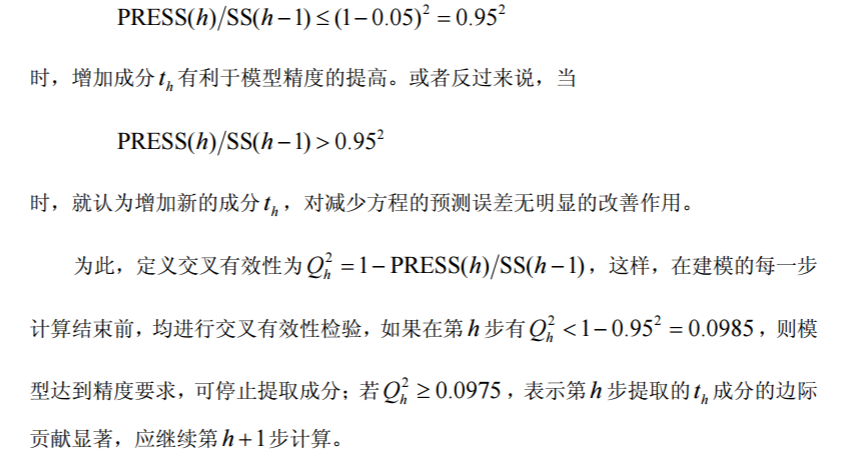偏最小二乘回归（二）：一种更简洁的计算方法

偏最小二乘回归（三）：身体特征与体能训练结果的 案例分析

展开全文• 偏最小二乘回归提供一种多对多线性回归建模的方法，特别当两组变量的个数很多，且都存在多重相关性，而观测数据的数量（样本量）又较少时，用偏最小二乘回归建立的模型具有传统的经典回归分析等方法所没有的优点。...
偏最小二乘回归提供一种多对多线性回归建模的方法，特别当两组变量的个数很多，且都存在多重相关性，而观测数据的数量（样本量）又较少时，用偏最小二乘回归建立的模型具有传统的经典回归分析等方法所没有的优点。偏最小二乘回归分析在建模过程中集中了主成分分析，典型相关分析和线性回归分析方法的特点，因此在分析结果中，除了可以提供一个更为合理的回归模型外，还可以同时完成一些类似于主成分分析和典型相关分析的研究内容，提供更丰富、深入的一些信息。

例：采用兰纳胡德（Linnerud）给出的关于体能训练的数据进行偏最小二乘回归建模。在这个数据系统中被测的样本点，是某健身俱乐部的20 位中年男子。被测变量分为两组。第一组是身体特征指标X ，包括：体重、腰围、脉搏。第二组变量是训练结果指标Y ，包括：单杠、弯曲、跳高。原始数据见表1。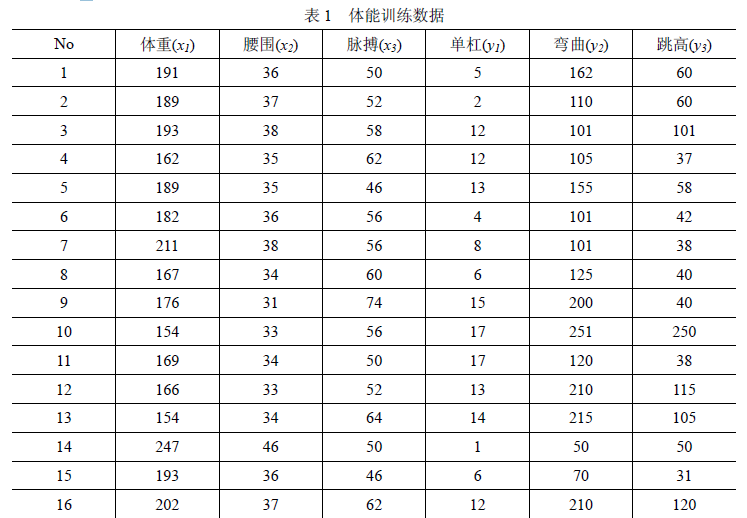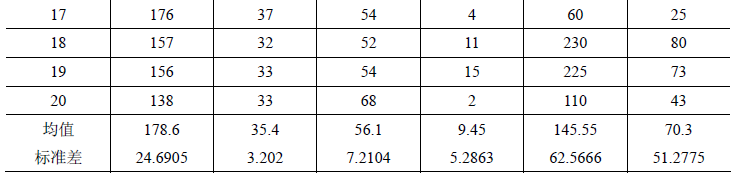表 2 给出了这6 个变量的简单相关系数矩阵。从相关系数矩阵可以看出，体重与腰围是正相关的；体重、腰围与脉搏负相关；而在单杠、弯曲与跳高之间是正相关的。从两组变量间的关系看，单杠、弯曲和跳高的训练成绩与体重、腰围负相关，与脉搏正相
关。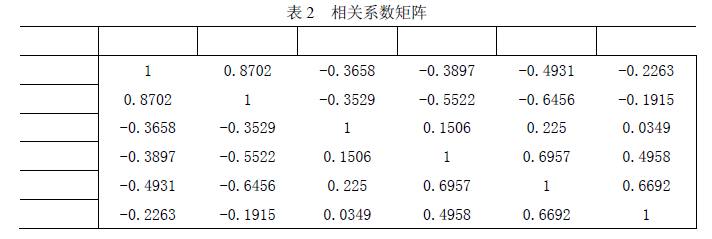MATLAB 程序：

clc,clear
mu=mean(pz);sig=std(pz); %求均值和标准差
rr=corrcoef(pz); %求相关系数矩阵
data=zscore(pz); %数据标准化
n=3;m=3; %n 是自变量的个数,m 是因变量的个数
x0=pz(:,1:n);y0=pz(:,n+1:end);
e0=data(:,1:n);f0=data(:,n+1:end);
num=size(e0,1);%求样本点的个数
chg=eye(n); %w 到w*变换矩阵的初始化
for i=1:n
%以下计算w，w*和t 的得分向量，
matrix=e0'*f0*f0'*e0;
[vec,val]=eig(matrix); %求特征值和特征向量
val=diag(val); %提出对角线元素
[val,ind]=sort(val,'descend');
w(:,i)=vec(:,ind(1)); %提出最大特征值对应的特征向量
w_star(:,i)=chg*w(:,i); %计算w*的取值
t(:,i)=e0*w(:,i); %计算成分ti 的得分
alpha=e0'*t(:,i)/(t(:,i)'*t(:,i)); %计算alpha_i
chg=chg*(eye(n)-w(:,i)*alpha'); %计算w 到w*的变换矩阵
e=e0-t(:,i)*alpha'; %计算残差矩阵
e0=e;
%以下计算ss(i)的值
beta=[t(:,1:i),ones(num,1)]\f0; %求回归方程的系数
beta(end,:)=[]; %删除回归分析的常数项
cancha=f0-t(:,1:i)*beta; %求残差矩阵
ss(i)=sum(sum(cancha.^2)); %求误差平方和
%以下计算press(i)
for j=1:num
t1=t(:,1:i);f1=f0;
she_t=t1(j,:);she_f=f1(j,:); %把舍去的第j 个样本点保存起来
t1(j,:)=[];f1(j,:)=[]; %删除第j 个观测值
beta1=[t1,ones(num-1,1)]\f1; %求回归分析的系数
beta1(end,:)=[]; %删除回归分析的常数项
cancha=she_f-she_t*beta1; %求残差向量
press_i(j)=sum(cancha.^2);
end
press(i)=sum(press_i);
if i>1
Q_h2(i)=1-press(i)/ss(i-1);
else
Q_h2(1)=1;
end
if Q_h2(i)<0.0975
fprintf('提出的成分个数r=%d',i);
r=i;
break
end
end
beta_z=[t(:,1:r),ones(num,1)]\f0; %求Y 关于t 的回归系数
beta_z(end,:)=[]; %删除常数项
xishu=w_star(:,1:r)*beta_z; %求Y关于X的回归系数，且是针对标准数据的回归系数，每一列是一个回归方程
mu_x=mu(1:n);mu_y=mu(n+1:end);
sig_x=sig(1:n);sig_y=sig(n+1:end);
for i=1:m
ch0(i)=mu_y(i)-mu_x./sig_x*sig_y(i)*xishu(:,i); %计算原始数据的回归方程的常数项
end
for i=1:m
xish(:,i)=xishu(:,i)./sig_x'*sig_y(i); %计算原始数据的回归方程的系数，每一列是一个回归方程
end
sol=[ch0;xish] %显示回归方程的系数，每一列是一个方程，每一列的第一个数是常数项
save mydata x0 y0 num xishu ch0 xish


展开全文• 　6.1　结构方程模型 　6.2　偏最小二乘通径模型 　6.3　多组变量集合的评估指数构建方法 　6.4　递阶偏最小二乘回归模型 　6.5　成分数据的线性回归分析方法 第7章 偏最小二乘回归的非线性方法 　7.1　引言　 　7.2...
• 采用偏最小二乘回归法获取电力负荷与各影响因素的关系方程；根据“十二五”及“十三五”期间经济和电力发展的特点，采用情景分析法设定多个情景及相应参数，获得不同情景下有区别的负荷预测结果，以降低预测风险。...
• 偏最小二乘回归： 多重共线性下，多变量对多变量的回归。本质上是对自变量做矩阵变换后的最小二乘回归。特点是矩阵变换的变换来源。
偏最小二乘回归
​

考虑自变量有多个，因变量只有一个时候的回归，当自变量的多重相关性差的时候，可以直接使用最小二乘求解回归模型；
考虑自变量有多个，因变量只有一个时候的回归，当自变量的多重共线性强的时候，可以对自变量做主成分分析，然后使用主成分作为新的自变量，再使用最小二乘求解回归模型；
考虑自变量有多个，因变量也有多个的时候的回归，如果自变量和因变量都不存在多重共线性的时候，可以分别使用自变量对每一个因变量做回归，使用最小二乘求解；
考虑自变量有多个，因变量也有多个的时候的回归，如果自变量存在多重共线性，因变量不存在多重共线性的时候，可以对自变量做主成分分析，然后使用主成分作为新的自变量分别对每一个因变量做回归，使用最小二乘求解；
考虑自变量有多个，因变量也有多个的时候的回归，如果自变量和因变量都存在多重共线性的时候该怎么办呢，这时候还是使用主成分分析+分别对每个变量做回归吗？
​
偏最小二乘提供一种多对多的线性回归建模方法，即自变量有多个，因变量也有多个的时候的建模方法，尤其适用于自变量和因变量都存在多重共线性的情况。
​
偏最小二乘在建模过程中集中了主成分分析、典型相关分析和线性回归分析方法的特点。
​

算法思想

主成分分析是求解组内方差最大的主成分，在求解主成分的时候，它控制了变换向量的范数；
典型相关分析是求解组间相关性最强的典型变量，在求解典型变量的时候，它控制了变换后的组内方差；
回归方程特点是能做回归预测，它是基于自变量数据输入得到因变量的预测值。
​
偏最小二乘回归最终是回归，它也是使用原始自变量变换后的变量做回归。它的变换是求解组间相关性最强的变量，不过它的约束条件是控制变换向量的范数。从形式上看，它使用了典型相关分析的目标函数和主成分分析的约束方程。另一个角度看，偏最小二乘的回归参数也是使用最小二乘估计的，所以它在回归参数求解的时候，对于多个因变量的参数是单独求解的。它的特点只是在于自变量的变换过程。
​
偏最小二乘最终的模型形式也是
$Y_{nq}=X_{np}\beta_{pq}+B$
​

算法流程
假设p个自变量$X_1,...,X_p$和q个因变量$Y_1,...,Y_q$。n个样本的标准化后的数据为$X_0(n*p),Y_0(n*q)$。

最佳变换向量求解以得到第一对成分。求解$w_1,v_1$使得$w_1^{'}X_0和v_1^{'}Y_0$的相关性最大，即协方差最大，对于标准化数据，也就是$w_1^{'}X_0和v_1^{'}Y_0$的内积最大，目标函数如下：
$\underset{\mid\mid w_1\mid\mid ^2 = 1,\mid\mid v_1\mid\mid ^2 = 1}{max}w_1^{'}X_0^{'}Y_0v_1$
目标函数可以使用拉格朗日乘子法求解，求解得到最佳的$w_1,v_1$，其中$w_1$称为模型效应权重，$v_1$称为因变量权重。以此得到变换后的成分$t_1=X_0w_1$
回归模型训练。根据上文得到的$t_1$，得到回归模型：
$\begin{cases}X_0=t_1\alpha_1^{'}+E_1\\ Y_0=t_1\beta_1^{'}+F_1\end{cases}$
使用最小二乘求解$\alpha_1和\beta_1$。
集成多个成分。因为$Y_0$是标准化后的变量，所以当模型效果好的时候，残差$F_1$中的元素绝对值应该近似为0。当使用单个成分的预测效果不够好怎么办呢？与Boost的加法模型思想相似，基于残差结果得到新的成分，以得到更优的模型。即，以$E_1=X_0-\hat{X_0},F_1=Y_0-\hat{Y_0}$重复步骤1和步骤2以得到第二个成分。此时得到的模型为：
$\begin{cases}X_0=t_1\alpha_1^{'}+t_2\alpha_2^{'}+E_2\\ Y_0=t_1\beta_1^{'}+t_2\beta_2^{'}+F_2\end{cases}$
以此类推，当$X_0$的秩为r<=min(n-1,p)时，存在r个成分$t_1,...,t_r$，使得：
$\begin{cases}X_0=t_1\alpha_1^{'}+...+t_r\alpha_r^{'}+E_r\\ Y_0=t_1\beta_1^{'}+...+t_r\beta_r^{'}+F_r\end{cases}$
模型整合。整合加法模型，将能提前相加的参数合并，得到最终的模型参数$\hat{\beta}$，模型为。
$\hat{Y}=X\hat{\beta}$
最优参数确定。偏最小二乘中确定成分的个数的方法是使用交叉验证的方式，即以交叉验证的方式确定最优的模型参数。
​

总结

偏最小二乘回归是一种使用预存在多重相关性的多组自变量、多组因变量的回归建模；
偏最小二乘是基于结合主成分分析和典型相关分析得到的成分变量的回归；
为什么使用交叉验证而不是像主成分分析一样使用累计贡献率呢？因为偏最小二乘中求解最佳变换向量的时候，并没有让组内方差最大，所以每个成分包含的组内信息大小不能确定，使用组内累计贡献率并没有意义。只能使用对因变量的预测效果来评估使用多少的主成分。不过因为成分的选取是逐个进行的，所以选择最佳成分的时候还是与主成分分析相同选取前$l$个。
​

思考

偏最小二乘方法计算过程中需要计算很多的特征值、特征向量，计算复杂度较大，方法不适用于大数据情况。从回归方程的思路看，可不可以单纯构造以下模型呢？
$\underset{W,\alpha,\beta}{min}\mid\mid X_0-X_0W\alpha\mid\mid ^2 + \gamma\mid\mid Y_0-X_0W\beta\mid\mid ^2$
其中W为一个p*k的矩阵，$\alpha$为一个k*p的矩阵，$\beta$为一个k*q的矩阵。k为人为设置的大于1，小于等于min(n-1,p)的超参，$\gamma$为人为设置的超参,$\mid\mid A\mid\mid$表示矩阵中所有元素的平方和。
​


展开全文统计学习 机器学习 多重共线性
• ## 偏最小二乘（pls）回归分析 matlab

万次阅读 多人点赞 2018-09-08 19:41:24
偏最小二乘用于查找两个矩阵（X和Y）的基本关系，即一个在这两个空间对协方差结构建模的隐变量方法。偏最小二乘模型将试图找到X空间的多维方向来解释Y空间方差最大的多维方向。偏最小二乘回归特别适合当预测矩阵比...
• 偏最小二乘回归提供一种多对多线性回归建模的方法，特别当两组变量的个数很多且都存在多重相关性，而观测数据的数量（样本量）又较少时，用偏最小二乘回归建立的模型具有传统的经典回归分析等方法所没有的优点。...
• 当因素很多且高度共线时，偏最小二乘（PLS）是一种构建预测模型的方法。它的重点在于预测响应，而不必试图理解变量之间的潜在关系。当预测是目标并且没有实际需要限制测量因子的数量时，PLS可能是有用的工具。 *PLS...机器学习
• 1、【分析】—【回归】—【权重估计】 ...可以自己该变幂的范围 3、得到的幂值，即m=1.5 4、【转换】—【计算变量】 ...6、然后出现此界面 ...9、观察系数，如图，得到加权最小二乘回归方程，即 ...经验分享
•matlab
• 在实际问题中，要研究两组多重相关变量间的相互依赖关系时，可使用的方法有：经典多元线性回归分析（MLR）、主成分回归分析（PCR）、偏最小二乘回归分析（PLS）。 当两组变量的个数很多，且都存在多重相关性，而观测...数学建模
• 偏最小二乘回归(PLS-Partial Least Squares)是一种新型的多元统计数据分析方法，是一种多因变量对多自变量的回归建模方法，是对最小二乘方法的推广。 优点： 1）提供了一种多因变量对多自变量的回归建模方法； 2）...机器学习 深度学习 数据挖掘 数学建模
• 当两组变量的个数很多，且都存在多重相关性，而观测数据的数量（样本量）又较少时，用偏最小二乘回归建立模型 11.1 分析概述 在自变量集中提出第一成分u（尽可能多的提取原自变量中的变异信息），同时在因变量集中...数学建模
• 小二乘回归提供一种多对多线性回归建模的方法，特别当两组变量的个数很多，且都存在多重相关性，而观测数据的数量（样本量）又较少时，用小二乘回归建立的模型具有传统的经典回归分析等方法所没有的优点。...
• 偏最小二乘回归： 我将围绕结构方程建模（SEM）技术进行一些咨询，以解决独特的业务问题。我们试图识别客户对各种产品的偏好，传统的回归是不够的，因为数据集的高度分量以及变量的多重共线性。PLS是处理这些有问题...R语言
• 应用偏最小二乘回归分析方法，以生物超微弱发光理论为依据，分析影响鱼类在繁殖阶段超微弱生物发光的主要因素，建立鱼类超微弱发光的预测方程，并阐述方程中各回归系数对应的物理意义，确定了影响鱼类超微弱生物发光...
• matlab做偏最小二乘  (2012-03-03 18:59:15 参考电脑中保存的《偏最小二乘回归.pdf》 clc,clear load ppz.txt %原始数据存放在纯文本文件pz.txt 中 pz=ppz; mu=mean(pz);sig=std(pz); %求均值和标准差 rr=...
•实例
• 8.4 对例5.5的Hald水泥问题用偏最小二乘方法建立模型，并与其他方法的结果进行比较。 x1 x2 x3 x4 y 7 26 6 60 78.5 1 29 15 52 74.3 11 56 8 20 104.3 11 31 8 47 87.6 7 52 6 33 95.9 11 55 9 22 109.2 3 71 17 6 ...
• 偏最小二乘回归 算法原理 给定p个自变量 和和q个因变量 ，各自有n个样本点，则自变量和因变量矩阵为 该算法的基本思想是，从原始变量中提取出K对潜在成份对tk和uk,k=1,2,...K；并通过潜在成分对数据...
• 偏最小二乘回归是一种回归形式 。 当使用pls时，新 的线性组合有助于解释模型中的自变量和因变量。 在本文中，我们将使用pls在“ Mroz”数据集中使用预测“收入”。 library(pls);library(Ecdat) data("Mroz")...R语言
• 偏最小二乘回归（一）：模型介绍 偏最小二乘回归（二）：一种更简洁的计算方法 本节采用兰纳胡德（Linnerud）给出的关于体能训练的数据进行偏最小二乘回归建 模。在这个数据系统中被测的样本点，是某健身俱乐部的 ...
• 偏最小二乘回归是一种新的多元统计数学分析方法，将多元线性回归、典型相关分析和主成分分析进行有机结合。 建模原理： 假定p有自变量x1,x2,…,xp（这个就是特征值）和q个因变量y1,y2,…,y3（这个就是你要预测的值）...
• MATLAB，可直接替换数据运行。主成分回归分析 Principal Component Regression（PCR）是一种多元回归分析方法，旨在解决自变量间存在多重共线性问题。PCA...